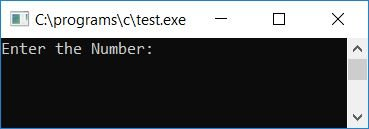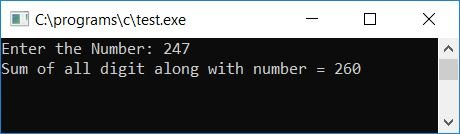# C program to add the digits of a number and the number itself

To add all the digits of any number along with the number itself in C programming, you have to ask the user to enter the number, add their digits along with the number itself, and then display the result on the output screen.

Let's suppose that the user has given 247 as input. Then, the program will calculate the sum of all the digits in the given number, and the number itself, that is, 2+4+7 or 13, will be again added to the number itself, that is, 13+247 or 260, which will be the final output as in the case of 247.

## Program to Add Number's Digits and Number in C

The question is: write a program in C that will ask the user to enter any number, and then this program has to find the sum of all the digits of that number along with the number itself. For example, if the number is 23, then 2  +  3  +  23 equals 260, which will be the output.

The following C program asks the user to enter any number and adds the digits of that number along with the number itself:

```#include<stdio.h>
#include<conio.h>
int main()
{
int num, rem=0, sum=0, temp;
printf("Enter the Number: ");
scanf("%d", &num);
temp = num;
while(num>0)
{
rem = num%10;
sum = sum+rem;
num = num/10;
}
sum = sum+temp;
printf("Sum of all digit along with number = %d", sum);
getch();
return 0;
}```

As the above programme was written in the Code::Blocks IDE, here is the sample run after a successful build and run. This is the first snapshot of the sample run:Now supply any number, say 247, to calculate the sum of digits (2+4+7) along with the number itself, that is, 2+4+7+247. Here is the second snapshot of the sample run:Here are some of the main steps used in the above program:

• Receive any number as input, say 247.
• Initialize this number to a variable, say temp.
• Create a while loop that runs until the number is greater than 0.
• Inside the while loop, find the last digit of the number using the modulous or remainder operator and add the digit one by one. Divide the number by 10 to check and enter into the loop again to find the sum of the next digit.
• That is, at first run, num, or 247, is greater than 0, therefore program flow goes inside the loop, and num%10, or 7, will be initialised to rem. Then sum+rem or 7 will be initialised to sum (never forget to initialise 0 to sum at the start of the program). Finally, divide the number by 10, that is, num/10, 247/10, or 24 will be initialised to num. After this, let's again check whether the value of num is greater than 0 or not.
• If it is, then again, program flow goes inside the while loop and does the same operation as done earlier.
• In this way, we can have a variable called "sum" that will hold the value of the sum of all the digits of the given number.
• Now add this to the number itself; that is, we have the value of the number initialised to the temp variable before it goes into the while loop.
• After summing, print the sum variable's value as output, which is 2 + 4 + 7 + 247, or 260, on the output screen.

C Quiz

« Previous Program Next Program »